GeeksforGeeks App
Open AppBrowser
Continue

# Surface Area of a Prism Formula

In mathematics, a prism is an essential member of the polyhedron family and is defined as a three-dimensional shape having two identical polygons facing each other that are connected by rectangular or parallelogram faces laterally. The identical polygons can be triangles, squares, rectangles, pentagons, or any other n-sided polygon and are called the bases of the prism. The other faces of a prism are parallelograms or rectangles. There are two kinds of prisms based on the type of the polygonal base, namely a regular prism and an irregular prism. Based on the alignment of the bases, there are two kinds of prisms, namely, the right prism and an oblique prism. Also, there are different types of prisms based on the shape of the base of a prism, such as

• Triangular prisms,
• Square prisms,
• Rectangular prisms,
• Pentagonal prisms,
• Hexagonal prisms,
• Octagonal prisms, etc.

### Surface Area of Prism

The surface area of a prism is referred to as the total area enclosed by all its faces. To determine a prism’s surface area, we must calculate the areas of each of its faces, then add the resulting areas. A prism has two kinds of surface areas, namely the lateral surface area and the total surface area.  The area occupied by a prism’s faces, excluding the two parallel faces (bases of a prism), is referred to as its lateral surface area.

#### The lateral surface area of a prism = [Base perimeter × height] square units

Now, a prism’s total surface area is the sum of the areas of its two bases and its lateral surface area.

The general formula for calculating the total surface area of any type of right prism is:

### Triangular Prism

A prism with a triangular base is referred to as a triangular prism. A triangular prism consists of three inclined rectangular surfaces and two parallel triangle bases. Let “H” be the height of the triangular prism; “a, b, and c” are the sides’ lengths, and “h” is the height of the triangular bases.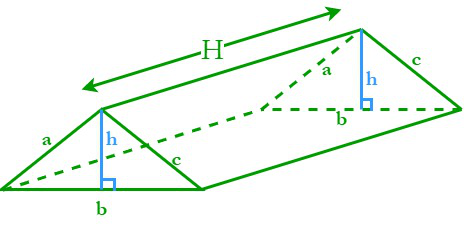The perimeter of a triangular base (P) = Sum of its three sides = a + b + c

The area of a triangular base (A) = ½ × base × height =  ½ bh

We know that the general formula for the lateral surface area of a right prism is L. S. A. = PH, where P is the base perimeter, and A is the base area.

By substituting all the values in the general formula we get,

#### The lateral surface area of a triangular prism = (a + b +c)H square units

where,

a, b, c are sides of triangular base

H is height of  triangular prism

We know that the general formula for the total surface area of a right prism is T. S. A. = PH+2A, where P is the base perimeter, A is the base area, and H is the height of the prism.

By substituting all the values in the general formula we get

The total surface area of the triangular prism = (a + b + c)H + 2 × (½ bh)

#### The total surface area of the triangular prism = (a + b + c)H + bh square units

where,

a, b, c are sides of triangular base

H is height of  triangular prism

h is height of triangle

### Rectangular Prism

A prism with a rectangular base is referred to as a rectangular prism. A rectangular prism consists of four rectangular surfaces and two parallel rectangular bases. Let the prism’s height be “h” and its rectangular bases’ length and width be “l” and “w,” respectively.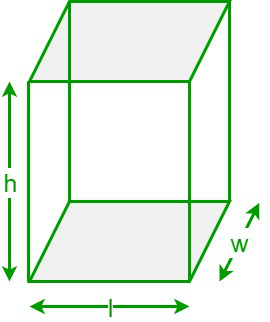The perimeter of a rectangular base (P) = Sum of its four sides = 2 (l + w)

The area of a rectangular base (A) = length × width =  l × w

We know that the general formula for the lateral surface area of a right prism is L. S. A. = PH, where P is the base perimeter, and A is the base area.

By substituting all the values in the general formula we get,

#### The lateral surface area of a rectangular prism = 2h(l + w) square units

where,

l is length

w is width

h is height

We know that the general formula for the total surface area of a right prism is T. S. A. = PH+2A, where P is the base perimeter, A is the base area, and H is the height of the prism.

By substituting all the values in the general formula we get

The total surface area of the rectangular prism = 2h(l + w) + 2(l × w)

= 2 lh + 2 wh + 2 lw

where,

l is length

w is width

h is height

### Square Prism

A prism with a square base is referred to as a square prism. A square prism consists of four rectangular surfaces and two parallel square bases. Let the prism’s height be “h” and its square bases’ lengths be “s”.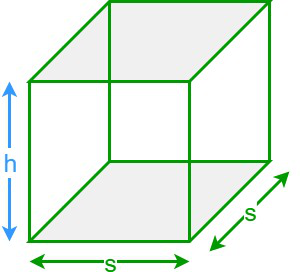The perimeter of a square base (P) = Sum of its four sides = s + s + s + s = 4s

The area of a square base (A) = (length of the side)2 =  s2

We know that the general formula for the lateral surface area of a right prism is L. S. A. = PH, where P is the base perimeter, and A is the base area.

By substituting all the values in the general formula we get,

#### The lateral surface area of a square prism = 4sh square units

where,

s is side of square base

h is height of square prism

We know that the general formula for the total surface area of a right prism is T. S. A. = PH+2A, where P is the base perimeter, A is the base area, and H is the height of the prism.

By substituting all the values in the general formula we get

#### The total surface area of the square prism = [4sh + 2s2] square units

where,

s is side of square base

h is height of square prism

### Pentagonal Prism

A prism with a pentagonal base is referred to as a pentagonal prism. A pentagonal prism consists of five inclined rectangular surfaces and two parallel pentagonal bases. Let “h” be the height of the pentagonal prism; “a and b” be the apothem length and side lengths of the pentagonal bases.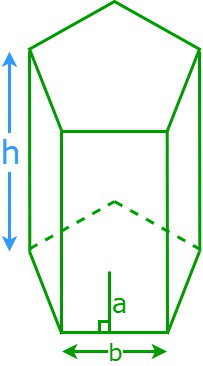The perimeter of a pentagon base (P) = Sum of its five sides = 5b

The area of a pentagon base (A) = 5/2 x (apothem length) x (length of the side) = 5ab

We know that the general formula for the lateral surface area of a right prism is L. S. A. = PH, where P is the base perimeter, and A is the base area.

By substituting all the values in the general formula we get,

#### The lateral surface area of a pentagonal prism = 5bh square units

where,

b is side of pentagonal base

h is the height of pentagonal prism

We know that the general formula for the total surface area of a right prism is T. S. A. = PH+2A, where P is the base perimeter, A is the base area, and H is the height of the prism.

By substituting all the values in the general formula we get,

#### The total surface area of the pentagonal prism = [5bh + 5ab] square units

where,

b is side of pentagonal base

a is apothem length.

h is the height of pentagonal prism

#### Hexagonal Prism

A prism with a hexagonal base is referred to as a hexagonal prism. A hexagonal prism consists of six inclined rectangular surfaces and two parallel hexagonal bases. Let “h” be the height of the hexagonal prism; “a” be the side lengths of the hexagonal bases.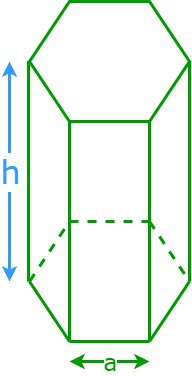The perimeter of a hexagon base (P) = Sum of its six sides = 6a

The area of a hexagon base (A) = 6 x (Area of an equilateral triangle)

A = 6 x (√3a2/4) ⇒  A = 3√3a2/2

We know that the general formula for the lateral surface area of a right prism is L. S. A. = PH, where P is the base perimeter, and A is the base area.

By substituting all the values in the general formula we get,

#### The lateral surface area of a hexagonal prism = 6ah square units

where,

a is side of hexagonal base

h is height of hexagonal base

We know that the general formula for the total surface area of a right prism is T. S. A. = PH+2A, where P is the base perimeter, A is the base area, and H is the height of the prism.

By substituting all the values in the general formula we get

#### The total surface area of the hexagonal prism =  [6ah +3√3a2 ]  square units

where,

a is side of hexagonal base

h is height of hexagonal base

### Sample Problems

Problem 1: What is the height of a prism whose base area is 36 square units, its base perimeter is 24 units, and its total surface area is 320 square units?

Solution:

Given data,

Base area = 36 square units

Base perimeter = 24 units

The total surface area of the prism = 320 square units

We have,

The total surface area of the prism = (2 × Base Area) + (Base perimeter × height)

⇒ 320 = (2 × 36)+ (24 × h)

⇒ 24h = 248 ⇒ h = 10.34 units

Hence, the height of the given prism is 10.34 units.

Problem 2: Find the total surface area of a square prism if the height of the prism and the length of the side of the square base are 13 cm and 4 cm, respectively.

Solution:

Given data,

The height of the square prism (h) = 13 cm

The length of the side of the square base (a) = 4 cm

We know that,

The total surface area of a square prism = 2a2 + 4ah

= 2 × (4)2 + 4 × 4 × 13

= 32 + 208 = 240 cm2

Hence, the total surface area of the given prism is 240 sq. cm.

Problem 3: Determine the base length of a pentagonal prism if its total area is 100 square units and its height and apothem length are 8 units and 5 units, respectively.

Solution:

Given data,

The total surface area of the pentagonal prism = 100 square units

The height of the prism (h) = 8 units

Apothem length (a) = 5 units

We know that,

The total surface area of the pentagonal prism = 5ab + 5bh

⇒ 100 = 5b (a+ h)

⇒ 100/5 = b (5 + 8)

⇒ 20 = b × (13) ⇒ b = 25/16 = 1.54 units

Hence, the base length is 1.54 units

Problem 4: Determine the height of the rectangular prism and the total area of a rectangular prism if its lateral surface area is 540 sq. cm and the length and breadth of the base are 13 cm and 7 cm, respectively.

Solution:

Given data,

The length of the rectangular base (l) = 13 cm

The width of the rectangular base (w) = 7 cm

The lateral surface area of the prism = 540 sq. cm

We have,

The lateral surface area of the prism = Base perimeter × height

⇒ 540 = 2 (l + w) h

⇒ 2 (13 + 7) h = 540

⇒ 2 (20) h = 540 ⇒ h = 13.5 cm

We know that,

The total surface area of the rectangular prism = 2 (lw + wh + lh)

= 2 × (13 × 7 + 7 × (13.5) + 13 × (13.5))

= 2 × (91 + 94.5 + 175.5) = 722 sq. cm

Hence, the height and total surface area of the given rectangular prism are 13.5 cm and 722 sq. cm, respectively.

Problem 5: Determine the surface area of the regular hexagonal prism if the height of the prism is 12 in and the length of the side of the base is 5 in.

Solution:

Given data,

The height of the prism (h) = 12 in

The length of the side of the base (a) = 6 in

The surface area of a regular hexagonal prism = 6ah + 3√3a2

= 6 × 5 × 12 + 3√3(5)2

= 360 + 75√3

= 360 + 75 × (1.732) = 489.9 sq. in

Hence, the surface area of the given prism is 489.9 sq. in.

Problem 6: Calculate the lateral and total surface areas of a triangular prism whose base perimeter is 25 inches, the base length and height of the triangle are 9 inches and 10 inches, and the height of the prism is 14 inches.

Solution:

Given data,

The height of the prism (H) = 14 inches

The base perimeter of the prism (P) = 25 inches

The base length of the triangle = 9 inches

The height of the triangle = 10 inches

We know that,

The lateral surface area of the prism = Base perimeter × height

= 25 × 14= 350 sq. in

Area of the triangular base (A) = ½ × base × height = 1/2 × 9 × 10 = 45 sq. in

The total surface area of the triangular prism = 2A + PH

= 2 × 45 + 25 × 14 = 90 + 350 = 440 sq. in

Hence, the prism’s lateral and total surface areas are 350 sq. in and 440 sq. in, respectively.

My Personal Notes arrow_drop_up
Related Tutorials